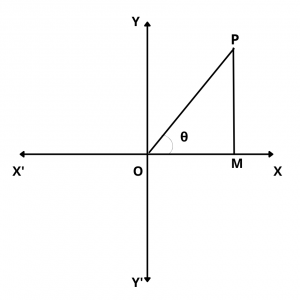# What is the General Solution of $$Cot \theta$$ = 0 ?

## Solution :

The general solution of $$cot \theta$$ = 0 is given by $$\theta$$ = $$(2n + 1){\pi\over 2}$$, n $$\in$$ Z.

Proof :

We have,$$cot \theta$$ = $$OM\over PM$$

$$\therefore$$   $$cot \theta$$ = 0

$$\implies$$  $$OM\over PM$$ = 0

$$\implies$$ OM = 0

$$\implies$$  OP coincides with OY or OY’

$$\implies$$  $$\theta$$ = $$\pm{\pi\over 2}$$, $$\pm{3\pi\over 2}$$, $$\pm{5\pi\over 2}$$, …….

$$\implies$$ $$\theta$$ = $$(2n + 1){\pi\over 2}$$,  n $$\in$$ Z

Hence, $$\theta$$ = $$(2n + 1){\pi\over 2}$$,  n $$\in$$ Z is the general solution of $$cot \theta$$ = 0.